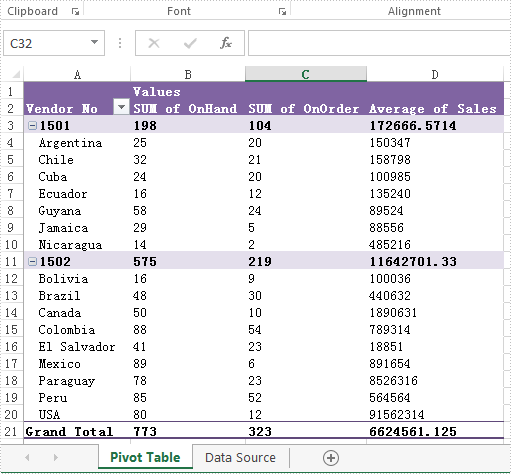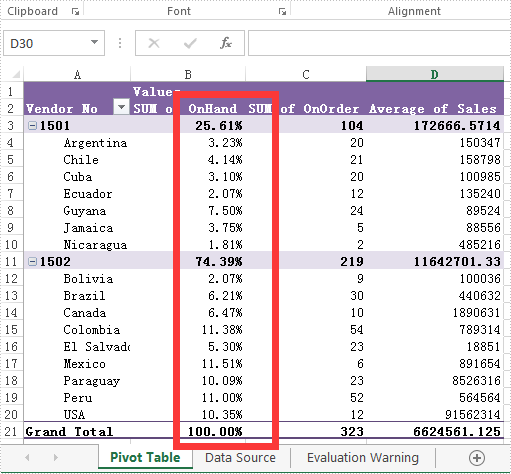# Set Data fields format on Excel PivotTable in C#

We have already demonstrated how to create the excel pivot table with Spire.XLS for .NET. It enables developers to set the property of PivotFieldFormatType to set format for the Data fields on pivot table. The following code sample will show you how to set display formats for data fields in C#.

Firstly please check the original DataField format on PivotTable:Step 1: Create a new Excel workbook and load from file.

```Workbook workbook = new Workbook();
```

Step 2: Get the first worksheet from the workbook.

```Worksheet sheet = workbook.Worksheets;
```

Step 3: Accessing the first Pivot table from the first worksheet.

```XlsPivotTable pt = sheet.PivotTables as XlsPivotTable;
```

Step 4: Accessing the Data Field.

```PivotDataField pivotDataField = pt.DataFields;
```

Step 5: Setting data display format by setting the property of PivotFieldFormatType as PercentageOfColumn.

```pivotDataField.ShowDataAs = PivotFieldFormatType.PercentageOfColumn;
```

Step 6: Save the document to file.

```workbook.SaveToFile("Result.xlsx", ExcelVersion.Version2010);
```

The effective screenshot after setting the Datafield format in PivotTable:Full codes of how to set the Datafields type in Excel Pivot Table.

```static void Main(string[] args)

{
Workbook workbook = new Workbook();

Worksheet sheet = workbook.Worksheets;

XlsPivotTable pt = sheet.PivotTables as XlsPivotTable;

PivotDataField pivotDataField = pt.DataFields;

pivotDataField.ShowDataAs = PivotFieldFormatType.PercentageOfColumn;

workbook.SaveToFile("Result.xlsx", ExcelVersion.Version2010);

}
```